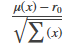# Efficient Portfolio That Maximizes Sharpe Ratio

Portfolios that maximize the Sharpe ratio are portfolios on the efficient frontier that satisfy several theoretical conditions in finance. Such portfolios are called tangency portfolios since the tangent line from the risk-free rate to the efficient frontier taps the efficient frontier at portfolios that maximize the Sharpe ratio.

The Sharpe ratio is defined as the ratiowhere $\mathit{x}\in {\mathit{R}}_{}^{\mathit{n}}$and ${\mathit{r}}_{0}^{}$is the risk-free rate ($\mu$ and $\Sigma$ proxies for the portfolio return and risk). For more information, see Portfolio Optimization Theory.

To obtain efficient portfolios that maximizes the Sharpe ratio, the `estimateMaxSharpeRatio` function accepts a `Portfolio` object and obtains efficient portfolios that maximize the Sharpe Ratio. Suppose that you have a universe with four risky assets and a riskless asset and you want to obtain a portfolio that maximizes the Sharpe ratio, where, in this example, ${\mathit{r}}_{0}^{}$ is the return for the riskless asset.

```r0 = 0.03; m = [ 0.05; 0.1; 0.12; 0.18 ]; C = [ 0.0064 0.00408 0.00192 0; 0.00408 0.0289 0.0204 0.0119; 0.00192 0.0204 0.0576 0.0336; 0 0.0119 0.0336 0.1225 ]; p = Portfolio('RiskFreeRate', r0); p = setAssetMoments(p, m, C); p = setDefaultConstraints(p); pwgt = estimateMaxSharpeRatio(p); display(pwgt)```
```pwgt = 4×1 0.4251 0.2917 0.0856 0.1977 ```

If you start with an initial portfolio, `estimateMaxSharpeRatio` also returns purchases and sales to get from your initial portfolio to the portfolio that maximizes the Sharpe ratio. For example, given an initial portfolio in `pwgt0`, you can obtain purchases and sales:

```pwgt0 = [ 0.3; 0.3; 0.2; 0.1 ]; p = setInitPort(p, pwgt0); [pwgt, pbuy, psell] = estimateMaxSharpeRatio(p); display(pwgt)```
```pwgt = 4×1 0.4251 0.2917 0.0856 0.1977 ```
`display(pbuy)`
```pbuy = 4×1 0.1251 0 0 0.0977 ```
`display(psell)`
```psell = 4×1 0 0.0083 0.1144 0 ```

If you do not specify an initial portfolio, the purchase and sale weights assume that you initial portfolio is `0`.